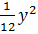### Sample Problem

What is the equation of a parabola with vertex at the origin and a directrix of x = -3?

(A) x =(B) x =(C) y =(D) y =#### Solution

x = - p

x = -3

p = 3

4xp = y2

4(3)x = y2

12x = y2

x =